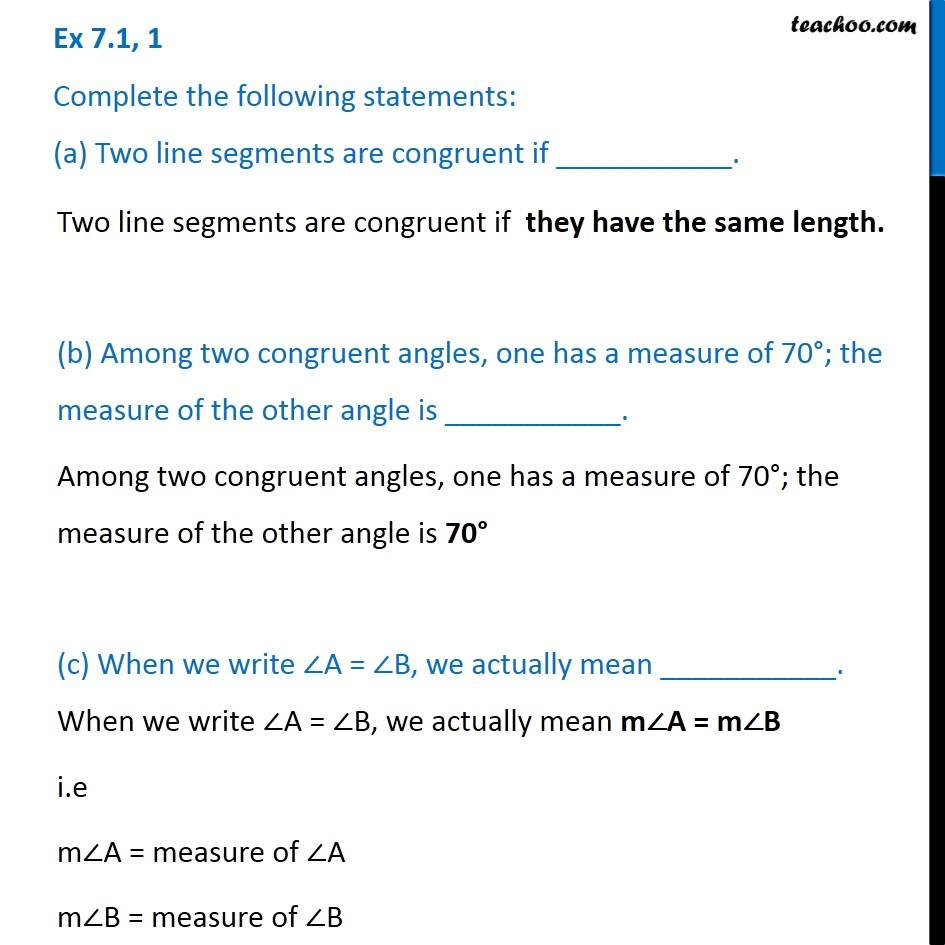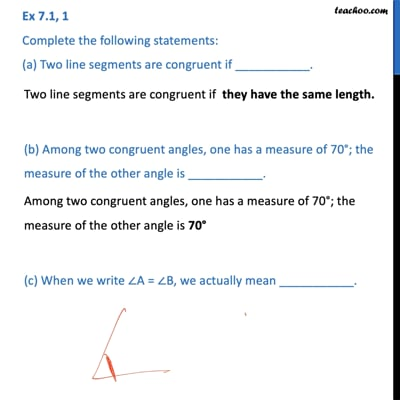Congruency - Definition

Congruence of Triangles
Serial order wiseThis video is only available for Teachoo black users

Learn in your speed, with individual attention - Teachoo Maths 1-on-1 Class

### Transcript

Question 1 Complete the following statements: (a) Two line segments are congruent if ___________. Two line segments are congruent if they have the same length. (b) Among two congruent angles, one has a measure of 70°; the measure of the other angle is ___________. Among two congruent angles, one has a measure of 70°; the measure of the other angle is 70° (c) When we write ∠A = ∠B, we actually mean ___________. When we write ∠A = ∠B, we actually mean m∠A = m∠B i.e m∠A = measure of ∠A m∠B = measure of ∠B